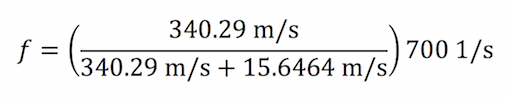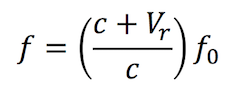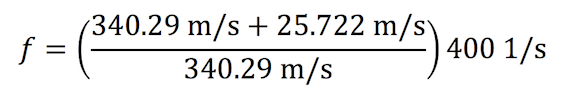Thursday, November 23, 2023
HomeEducationThe Doppler Effect Equation

# The Doppler Effect Equation

Doppler Effect Equation: What happens when an ambulance speeds past you? How does the sound of the siren change? It does get louder as it approaches, but another characteristic of sound changes also. The pitch is higher when it moves towards you (think a whining noise), and lower when it moves away (think a deep voice). This change in pitch has to do with the frequency of the waves, or how many waves pass through an area per unit time.

In the case of the ambulance, you are standing still and the ambulance is approaching you. Since the sound waves are moving towards you, they are compressed and the frequency increases, and thus you hear a higher pitch. However, when the ambulance moves away from you, the sound waves are spread further apart and the frequency gets lower, so you hear a lower pitch. This change in sound wave frequency due to movement is called the Doppler shift, also known as the Doppler effect.

The Doppler effect is not all theoretical though. We can use the Doppler effect equation to calculate both the velocity of the source and observer, the original frequency of the sound waves, and the observed frequency of the sound waves.

## The Doppler Effect Equation

A common example of Doppler shift is the change of pitch heard when a vehicle sounding a horn approaches and recedes from an observer. Compared to the emitted frequency, the received frequency is higher during the approach, identical at the instant of passing by and lower during the recession.

The reason for the Doppler effect is that when the source of the waves is moving towards the observer, each successive wave crest is emitted from a position closer to the observer than the crest of the previous wave. Therefore, each wave takes slightly less time to reach the observer than the previous wave. Hence, the time between the arrivals of successive wave crests at the observer is reduced, causing an increase in the frequency.

##### While they are traveling, the distance between successive wavefronts is reduced, so the waves “bunch together”.

Conversely, if the source of waves is moving away from the observer, each wave is emitted from a position farther from the observer than the previous wave, so the arrival time between successive waves is increased, reducing the frequency. The distance between successive wavefronts is then increased, so the waves “spread out”.

For waves that propagate in a medium, such as sound waves, the velocity of the observer and of the source are relative to the medium in which the waves are transmitted. The total Doppler effect may, therefore, result from the motion of the source, motion of the observer, or motion of the medium. Each of these effects is analyzed separately. For waves that do not require a medium, such as light or gravity in general relativity, only the relative difference in velocity between the observer and the source needs to be considered.

The Doppler Effect Equation & Calculating Frequency Change. … You listen as the ambulance draws near, and after it passes, the pitch, or frequency, of the siren seems to change. In 1842 Christian Doppler hypothesized that sound frequencies change, relative to the observer, when emitted from a moving sound source.

A common example of Doppler shift is the change of pitch heard when a vehicle sounding a horn approaches and recedes from an observer. Compared to the emitted frequency, the received frequency is higher during the approach, identical at the instant of passing by and lower during the recession.

When the distance is decreasing, the frequency of the received waveform will be higher than the source waveform. Besides sound and radio waves, the Doppler effect also affects the light emitted by other bodies in space. If a body in space is “blue-shifted,” its light waves are compacted and it is coming towards us.

## Doppler Effect Equation Light

The formula for determining the frequency during this event is as follows:ƒ = observed frequency

c = speed of sound

Vs = velocity of the source (negative if it’s moving toward the observer)

ƒ0 = emitted frequency of the source

Suppose you are standing on the corner of 5th Avenue and 34th Street waiting for the light to change so you can cross the street. An approaching southbound ambulance is heading your way traveling at 35 miles per hour. If we know that the frequency of the ambulance siren is 700 Hz, we can calculate the frequency of what you hear:

c, the speed of sound, is 340.29 m/s

Vs, the velocity of the source, is 35 mi/h, or 15.6464 m/s

ƒ0, the frequency of the ambulance siren, is 700 Hz

Therefore,becomes
(note: Vs is negative since the source is moving towards the observer)##### which reduces to:

ƒ ≈ 734 Hz

Once the ambulance passes, the frequency of the sound decreases, or sounds “lower”. The same calculation is performed to determine the observed frequency, except in this case Vs is positive:##### ƒ ≈ 669 Hz

A similar change in sound frequency is observed if the observer is moving towards a stationary sound source. In this case, the formula is:Where Vr is the velocity of the receiver or observer. (note: this is negative if the observer is moving away from the source).

So, as an example, if you are driving your boat at 50 knots towards a buoy with a foghorn emitting a 400 Hz signal, the frequency of the sound you hear would be:Where is Vr is 50 knots or 25.722 m/sƒ ≈ 430 Hz

We can also calculate the observed frequency if both the source sound and the observer are moving towards each other. In this case, the formula is:Now pretend that you and your friends are sitting on top of a tour bus heading southbound on 7th Avenue at 30 miles per hour. That same ambulance is heading towards your bus at 28 miles per hour. We can now calculate the frequency of what you hear:

Vr, the velocity of the source, is 30 mi/h, or 13.4112 m/s
Vs, the velocity of the source, is 28 mi/h, or 12.51712 m/sƒ ≈ 755 Hz

## Doppler Effect Equation Signs

So far, we have only considered stationary sources of sound and stationary listeners (or observers). However, if either the source or the observer is moving, things change. This is called the Doppler effect. Like the idea of feedback, covered in the last two sections, the Doppler effect has many important applications. Because the Doppler effect depends on things moving, it can generally be used to determine the motion or speed of an object. Objects of interest may be the speed of a car on the highway, the motion of blood flowing through an artery, the rotation of a galaxy, even the expansion of the Universe. As with many fundamental principles in physics, the range of applications can be truly enormous.

##### So, what is the Doppler effect? One of the most common examples is that of the pitch of a siren on an ambulance or a fire engine.

You may have noticed that as a fast-moving siren passes by you, the pitch of the siren abruptly drops in pitch. At first, the siren is coming towards you, when the pitch is higher. After passing you, the siren is going away from you and the pitch is lower. This is a manifestation of the Doppler effect.

Read Also: Reflexive Property Of Equality

There are two different situations for the Doppler effect that we will investigate. The first is where the observer is moving. For example, you are in a moving car and are passing by a stationary siren. In the other case, you are stationary, and the source is moving past you. While the second is perhaps the more common situation, the first is easier to analyze. You also might think that these two situations are the same. As it turns out, they are not and this means that you can also learn about who is moving, the source or the observer. We will return to this question in the next section.

## Equation For Doppler Effect

A Doppler shift is a phenomenon of a change in frequency based on the observers’ point of view. The most common analogy of this is standing on the side of the road and listen to a passing car. As the car approaches, there is a definitive sound. As the car passes, the sound changes to a lower frequency. This is called a Doppler Shift. There are two types of Doppler shifts:

• Red-Shift or a shift of frequency to a lower wavelength (away from the observer)
• Blue-Shift or a shift of frequency to a higher wavelength (toward the observer)

Anything that emits wavelengths – light, radio, gamma rays, and the rest of the E-M Band – and changes frequency due to movement, a Doppler shift can be measured. This is the equation: